# Bihar Board Class 10th Maths Solutions Chapter 12 Areas Related to Circles Ex 12.3

Bihar Board Class 10th Maths Solutions Chapter 12 Areas Related to Circles Ex 12.3 Textbook Questions and Answers.

## BSEB Bihar Board Class 10th Maths Solutions Chapter 12 Areas Related to Circles Ex 12.3Question 1.
Find the area of the shaded region in the figure, if PQ = 24 cm, PR = 7 cm and O is the centre of the circle.[Annual Paper (Delhi) 2009]
Solution:
Since ROQ is a diameter, therefore ∠RPQ = 90°.
RQ² = RP² + PQ²
or RQ² = 7² + 24² = 49 + 576 = 625
or RQ = $$\sqrt{625}$$ cm = 25 cm
∴ Radius r = $$\frac { 1 }{ 2 }$$ RQ = $$\frac { 25 }{ 2 }$$ cm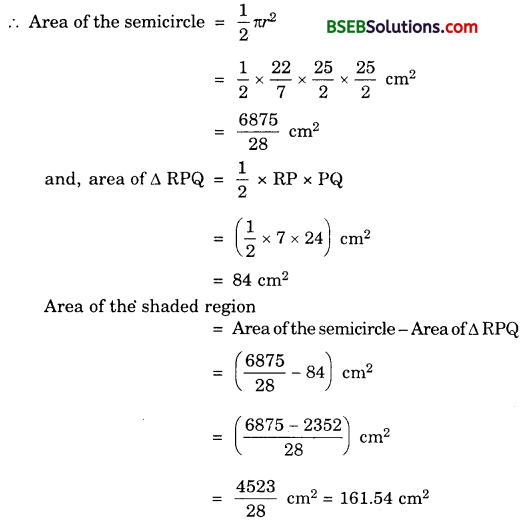Question 2.
Find the area of the shaded region in the figure, if radii of the two concentric circles with centre O are 7 cm and 14 cm respectively and ∠AOC = 40°.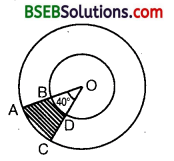Solution:
Area of the shaded region = Area of sector AOC – Area of sector OBD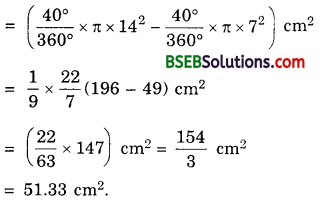Question 3.
Find the area of the shaded A region in the figure, if ABCD is a square of side 14 cm and APD and BPC are semicircles.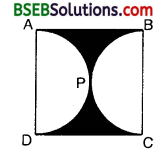Solution:
Area of the square ABCD = (14)² cm² = 196 cm²
Diameter of the semicircles = AD or BC = 14 cm
∴ Radius of each semicircle = $$\frac { 14 }{ 2 }$$ cm = 7 cm
∴ Area of the both semicircular regions
= 2 x $$\frac { 1 }{ 2 }$$πr² = πr²
= ($$\frac { 22 }{ 7 }$$ x 49) cm² = 154 cm²
∴ Area of the shaded regions = Area of the square ABCD – Area of the both semicircular regions
= (196 – 154) cm² – 42 cm².

Question 4.
Find the area of the shaded region in the figure, where a circular arc of radius 6 cm has been drawn with vertex O of an equilateral triangle OAB of side 12 cm as centre.
Solution:
Area of the circular portion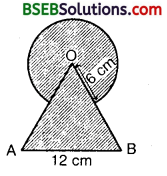Area of the equilateral ∆ OAB
$$\frac{\sqrt{3}}{4}$$(side)² = ($$\frac{\sqrt{3}}{4}$$ x 144) cm²
= 36$$\sqrt{3}$$ cm²
Area of the shaded region = Area of circular portion + Area of the equilateral triangle
= $$\left(\frac{660}{7}+36 \sqrt{3}\right)$$ cm²

Question 5.
From each corner of a square of side 4 cm a quadrant of a circle of radius 1 cm is cut and also a circle of diameter 2 cm is cut as shown in the figure. Find the area of the remaining portion of the square.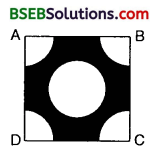Solution:
The area of the square ABCD = (side)²
= 4² cm²
= 16 cm²
The sum of the areas of the four quadrants at the four corners of the square
= The area of a circle of radius 1 cm
= $$\frac { 22 }{ 7 }$$ x 1² cm²
= $$\frac { 22 }{ 7 }$$ cm²
The area of the circle of diameter 2 cm, i.e., radius 1 cm²
= $$\frac { 22 }{ 7 }$$ x 1² cm²
= $$\frac { 22 }{ 7 }$$ cm²
Area of the remaining portion of the square = The area of the square ABCD – The sum of the areas of 4 quadrants at the four corners of the square – The area of the circle of diameter 2 cm
= (16 – $$\frac { 22 }{ 7 }$$ – $$\frac { 22 }{ 7 }$$) cm²
= $$\left(\frac{112-22-22}{7}\right)$$ cm²
= $$\frac { 68 }{ 7 }$$ cm² – 9.71 cm²Question 6.
In a circular table cover of radius 32 cm, a design is formed leaving an equilateral triangle ABC in the middle as shown in the figure. Find the area of the design (shaded region).Solution:
Let ABC be an equilateral triangle and let 0 be the circumcentre of the circumcircle of radius 32 cm.Draw OM ⊥ BC.
Now, ∠BOM = $$\frac { 1 }{ 2 }$$ x 120° = 60°
So, from ∆ BOM, we haveHence, area of ∆ BOC
= $$\frac { 1 }{ 2 }$$ BC x OM
= $$\frac { 1 }{ 2 }$$ x 32$$\sqrt{3}$$ x 16 cm²
Area of ∆ ABC = 3 x Area of ∆ BOC
= (3 x $$\frac { 1 }{ 2 }$$ x 32$$\sqrt{3}$$ x 16) cm²
= 768$$\sqrt{3}$$ cm²
∴ Area of the design (i.e., shaded region) = Area of the circle – Area of ∆ ABC
= $$\left(\frac{22528}{7}-768 \sqrt{3}\right)$$ cm²

Question 7.
In the figure, ABCD is a square of side 14 cm. With centres A, B, C and D, four circles are drawn such that each circle touch externally two of the remaining three circles. Find the area of the shaded region.Solution:
The area of the square ABCD = (side)² = 142 cm²
= 196 cm².
The sum of the areas of the four quadrants at the four corners of the square
= The area of a circle of radius $$\frac { 14 }{ 2 }$$ cm = 7 cm²
= π(7)² cm² =($$\frac { 22 }{ 7 }$$ x 49) cm² = 154 cm²
Area of the shaded region = The area of the square ABCD – The sum of the areas of four quadrants at the four corners of the square
= (196 – 154) cm² = 42 cm²Question 8.
Figure depicts a racing track whose left and right ends are semicircular.The distance between the two inner parallel line segments is 60 m and they are each 106 m long. If the track is 10 m wide, find
(i) the distance around the track along its inner edge.
(ii) the area of the track.
Solution:
We have OB = O’C = 30 m
and, AB = CD = 10 m
OA = O’D = (30 + 10) m = 40 m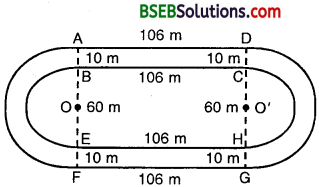(i) the distance around the track along its inner edge.
= BC + EH + 2 x circumference of the semicircle of radius OB = 30 m
= ( 106 + 106 + 2 x $$\frac { 1 }{ 2 }$$ x 2π(30) ) m
= ( 212 + 2 x $$\frac { 22 }{ 7 }$$ x 3o) m
= ( 212 + $$\frac { 1320 }{ 7 }$$ )m = $$\left(\frac{1484+1320}{7}\right)$$ m
= $$\frac { 2804 }{ 7 }$$ m
m = 400.57 m

(ii) Area of the track = Area of the shaded region = Area of rectangle ABCD + Area of rectangle EFGH + 2(Area of the semicircle of radius 40 m – Area of the semicircle with radius 30 m)Question 9.
In the figure, AB and CD are two diameters of a circle (with centre O) perpendicular to each other and OD is the diameter of the smaller circle. If OA = 7 cm, find the area of the shaded region.Solution:
Area of the sector OCB = $$\left(\frac{90^{\circ}}{360^{\circ}} \times \frac{22}{7} \times 7 \times 7\right)$$ cm²
= $$\frac { 77 }{ 2 }$$ cm²
Area of ∆ OCB = $$\frac { 1 }{ 2 }$$ x OC x OB
= $$\frac { 1 }{ 2 }$$ x 7 x 7 cm²
= $$\frac { 49 }{ 2 }$$ cm²
The area of the segment BPC = Area of the sector OCB – Area of the A OCB
= ($$\frac { 77 }{ 2 }$$ – $$\frac { 49 }{ 2 }$$) cm²
= $$\frac { 28 }{ 2 }$$ cm²
= 14 cm²
Similarly, the area of the segment AQC
= 14 cm²
Also,the area of the circle with DO as diameter
= ($$\frac { 22 }{ 7 }$$ x $$\frac { 7 }{ 2 }$$ x $$\frac { 7 }{ 2 }$$) cm² = $$\frac { 77 }{ 2 }$$ cm²
Hence,the total area of the shaded region
= (14 + 14 + $$\frac { 77 }{ 2 }$$) cm²
= $$\left(\frac{28+28+77}{2}\right)$$ cm²
= $$\frac { 133 }{ 2 }$$ cm²
= 66.5 cm²Question 10.
The area of an equilateral triangle ABC is 17320.5 cm². With each vertex of the triangle as centre, a circle is drawn with radius equal to half the length of the side of the triangle (see figure). Find the area of the shaded region.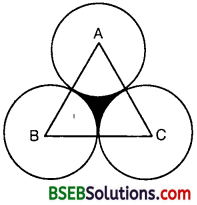(Use π = 3.14 and $$\sqrt{3}$$ = 1.73205)
Solution:
Let each side of the triangle be a cm. Then,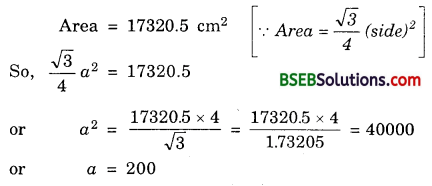Thus, radius of each circle is $$\frac { 200 }{ 2 }$$ cm = 100 cm.
= Area of ∆ ABC – 3 x (Area of a sector of angle 60° in a circle of radius 100 cm)
= [17320.5 – 3($$\frac{60^{\circ}}{360^{\circ}}$$ x 3.14 x 100 x 100)] cm²
= (17320.5 – 15700) cm² = 1620.5 cm²

Question 11.
On a square handkerchief, nine circular designs each of radius 7 cm are made (see figure). Find the area of the remaining portion of the handkerchief.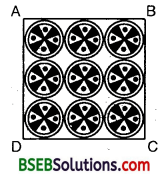Solution:
Side of the square ABCD
= AB – 3 x diameter of circular design
= 3 x (2 x 7) cm – 42 cm
∴ Area of the square ABCD
= (42 x 42) cm² = 1764 cm²
Area of one circular Resign
= πr² = ($$\frac { 22 }{ 7 }$$ x 7 x 7) cm²
= 154 cm²
∴ Area of 9 such designs
= (9 x 154) cm² = 1386 cm²
∴ Area of the remaining portion of the handkerchief
= Area of the square ABCD – Area of 9 circular designs
= (1764 – 1386) cm² – 378 cm²

Question 12.
In the figure, OACB is a quadrant of a circle with centre O and radius 3.5 cm. If OD = 2 cm, find the area of theSolution:Hence, area of the shaded region = Area of quadrant – Area of ∆ BOD
= ($$\frac { 77 }{ 8 }$$ – $$\frac { 7 }{ 2 }$$) cm² = ($$\frac { 77-28 }{ 8 }$$) cm²
= $$\frac { 49 }{ 8 }$$ cm² = 6.125 cm²Question 13.
In the figure, a square OABC is inscribed in a quadrant OPBQ. If OA = 20 cm, find the area of the shaded region. (Use π = 3.14)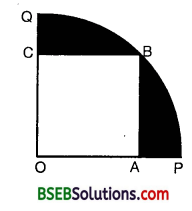Solution:
Radius of the quadrant = OB = $$\sqrt{\mathrm{OA}^{2}+\mathrm{AB}^{2}}$$
= $$\sqrt{20^{2}+20^{2}}$$ cm
= 20$$\sqrt{1+1}$$ cm
= 20$$\sqrt{2}$$ cm
= $$\frac { 1 }{ 4 }$$πr²
= $$\frac { 1 }{ 4 }$$ x 314 x (20$$\sqrt{2}$$)² cm²
= ( $$\frac { 1 }{ 4 }$$ x 3.14 x 80o) cm² = 628 cm²
Area of the square OABC = (20) cm² = 400 cm²
Hence, area of the shaded region = Area of quadrant – Area of square OABC
= (628 – 400) cm² = 228 cm².

Question 14.
AB and CD are respectively arcs of two concentric circles of radii 21 cm and 7 cm and centre O (see figure). If ∠AOB = 30°, find the area of the shaded region.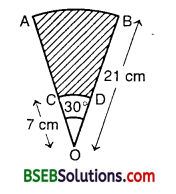Solution:
Let A1 and A2 be the areas of sector OAB and OCD respectively. Then,
A1 = Area of a sector of angle 30° in a circle of radius 21 cmA2 = Area of a sector of angle 30° in a circle of radius 7 cm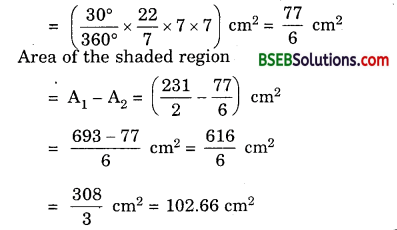Question 15.
In the figure, ABC is a quadrant of a circle of radius 14 cm and a semicircle is drawn with BC as diameter. Find the area of the shaded region.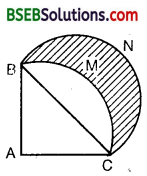[Annual Paper (Foreign) 2008]
Solution:
Here the radius of the quadrant with A as the centre is 14 cm.
Then, the area of the quadrant ABMC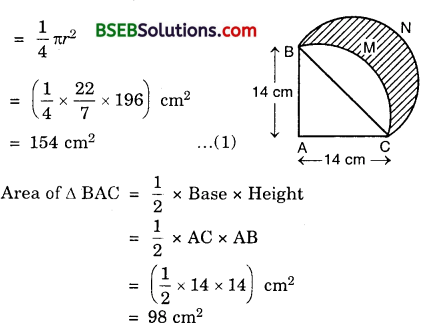Area of the segment BMC of the circle = Area of the quadrant ABMC – Area of ∆ BAC
= (154 – 98) cm²
= 56 cm²
Now, since AC = AB = 14 cm and ∠BAC = 90°, therefore by Pythagoras Theorem,
BC = $$\sqrt{\mathrm{AC}^{2}+\mathrm{AB}^{2}}$$
= $$\sqrt{14^{2}+14^{2}}$$
= 14$$\sqrt{2}$$ cm
∴ Radius of the semicircle BNC = $$\frac { 1 }{ 2 }$$ x 14$$\sqrt{2}$$ cm = 7$$\sqrt{2}$$ cm
∴ Area of the semicircle BNC
= (7$$\sqrt{2}$$ )² cm²
= $$\left(\frac{1}{2} \times \frac{22}{7} \times 98\right)$$ cm² = 154 cm²
Hence, the area of the shaded region
= the area of the region between two arcs BMC and BNC = The area of semicircle BNC – The area of the segment of the circle BMC
= (154 – 56) cm² = 98 cm²Question 16.
Calculate the area of the designed region in figure common between the two quadrants of circles of radius 8 cm each.Solution:
Here, 8 cm is the radius of each of the quadrants ABMD and BNDC.
Sum of their areas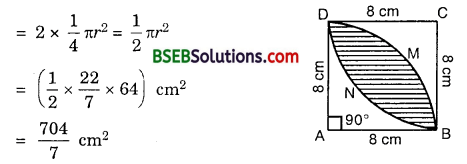Area of the square ABCD = (8 x 8) cm² = 64 cm²
Area of the designed region = Area of the shaded region
= Sum of the areas of quadrants – Area of the square ABCD
= $$\left(\frac{704}{7}-64\right)$$ cm² = $$\left(\frac{704-448}{7}\right)$$ cm²
= $$\frac{256}{7}$$ cm²
= 36.57 cm²